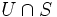Null subset of a differential manifold

Let$M$ be a differential manifold. A subset$S$ of$M$ is said to have measure zero or to be a null subset if the following holds: for any open subset$U$ of$M$ and any diffeomorphism between$U$ and$\R^n$, the image of$U \cap S$ under the diffeomorphism has measure zero in$\R^n$.
The well-definedness of this notion rests on the fact that any diffeomorphism between open subsets of$\R^n$ maps measure zero subsets to measure zero subsets (this is not true for arbitrary homeomorphisms, and hence the notion of a measure zero subset does not make sense for a topological manifold). Further information: Diffeomorphism implies nullset-preserving